## US Units of Measurement

### Learning Outcomes

• Define units of length and convert from one to another.
• Perform arithmetic calculations on units of length.
• Solve application problems involving units of length.
• Define units of weight and convert from one to another.
• Perform arithmetic calculations on units of weight.
• Solve application problems involving units of weight.
• Describe the general relationship between the U.S. customary units and metric units of length, weight/mass, and volume.
• Define the metric prefixes and use them to perform basic conversions among metric units.
• Solve application problems involving metric units of length, mass, and volume.
• State the freezing and boiling points of water on the Celsius and Fahrenheit temperature scales.
• Convert from one temperature scale to the other, using conversion formulas.

Measurement is a number that describes the size or amount of something. You can measure many things like length, area, capacity, weight, temperature and time. In the United States, two main systems of measurement are used: the metric system and the U.S. customary measurement system.

In this section we will explore units for length, weight, and capacity, as well as solve problems that involve converting between different units of length, weight or capacity.

## Units of Length

This topic addresses the measurement of length using the U.S. customary measurement system.

Suppose you want to purchase tubing for a project, and you see two signs in a hardware store: $1.88 for 2 feet of tubing and$5.49 for 3 yards of tubing. If both types of tubing will work equally well for your project, which is the better price? You need to know about two units of measurement, yards and feet, in order to determine the answer.

Length is the distance from one end of an object to the other end, or from one object to another. For example, the length of a letter-sized piece of paper is 11 inches. The system for measuring length in the United States is based on the four customary units of length: inch, foot, yard, and mile. Below are examples to show measurement in each of these units.

 Unit Description Image Inch/Inches Some people donate their hair to be made into wigs for cancer patients who have lost hair as a result of treatment. One company requires hair donations to be at least 8 inches long.Frame size of a bike: the distance from the center of the crank to the top of the seat tube. Frame size is usually measured in inches. This frame is 16 inches.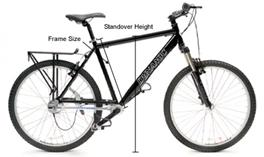Foot/Feet Rugs are typically sold in standard lengths. One typical size is a rug that is 8 feet wide and 11 feet long. This is often described as an 8 by 11 rug.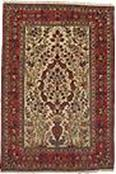Yard/Yards Soccer fields vary some in their size. An official field can be any length between 100 and 130 yards.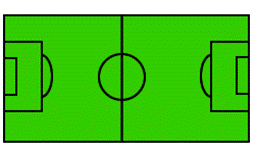Mile/Miles A marathon is 26.2 miles long. One marathon route is shown in the map to the right.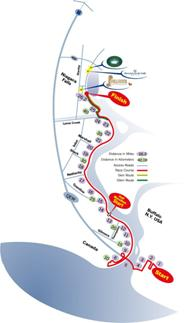You can use any of these four U.S. customary measurement units to describe the length of something, but it makes more sense to use certain units for certain purposes. For example, it makes more sense to describe the length of a rug in feet rather than miles, and to describe a marathon in miles rather than inches.

You may need to convert between units of measurement. For example, you might want to express your height using feet and inches (5 feet 4 inches) or using only inches (64 inches). You need to know the unit equivalents in order to make these conversions between units.

The table below shows equivalents and conversion factors for the four customary units of measurement of length.

 Unit Equivalents Conversion Factors (longer to shorter units of measurement) Conversion Factors (shorter to longer units of measurement) 1 foot = 12 inches $\displaystyle \frac{12\ \text{inches}}{1\ \text{foot}}$ $\displaystyle \frac{1\text{ foot}}{12\text{ inches}}$ 1 yard = 3 feet $\displaystyle \frac{3\text{ feet}}{1\text{ yard}}$ $\displaystyle \frac{\text{1 yard}}{\text{3 feet}}$ 1 mile = 5,280 feet $\displaystyle \frac{5,280\text{ feet}}{1\text{ mile}}$ $\displaystyle \frac{\text{1 mile}}{\text{5,280 feet}}$

Note that each of these conversion factors is a ratio of equal values, so each conversion factor equals 1. Multiplying a measurement by a conversion factor does not change the size of the measurement at all since it is the same as multiplying by 1; it just changes the units that you are using to measure.

### Convert Between Different Units of Length

You can use the conversion factors to convert a measurement, such as feet, to another type of measurement, such as inches.

Note that there are many more inches for a measurement than there are feet for the same measurement, as feet is a longer unit of measurement. You could use the conversion factor $\displaystyle \frac{\text{12 inches}}{\text{1 foot}}$.

If a length is measured in feet, and youd like to convert the length to yards, you can think, I am converting from a shorter unit to a longer one, so the length in yards will be less than the length in feet. You could use the conversion factor $\displaystyle \frac{\text{1 yard}}{\text{3 feet}}$.

If a distance is measured in miles, and you want to know how many feet it is, you can think, I am converting from a longer unit of measurement to a shorter one, so the number of feet would be greater than the number of miles. You could use the conversion factor $\displaystyle \frac{5,280\text{ feet}}{1\text{ mile}}$.

You can use the factor label method (also known as dimensional analysis) to convert a length from one unit of measure to another using the conversion factors. In the factor label method, you multiply by unit fractions to convert a measurement from one unit to another. Study the example below to see how the factor label method can be used to convert $\displaystyle 3\frac{1}{2}$ feet into an equivalent number of inches.

### Example

How many inches are in $\displaystyle 3\frac{1}{2}$ feet?

Notice that by using the factor label method you can cancel the units out of the problem, just as if they were numbers. You can only cancel if the unit being cancelled is in both the numerator and denominator of the fractions you are multiplying.

In the problem above, you cancelled feet and foot leaving you with inches, which is what you were trying to find.

$\frac{7}{2}\cancel{\text{feet}}\cdot\frac{12\text{ inches}}{\cancel{1\text{foot}}}=\text{? inches}$

What if you had used the wrong conversion factor?

$\frac{7}{2}\text{feet}\cdot\frac{1\text{foor}}{12\text{ inches}}=\text{? inches}$?

You could not cancel the feet because the unit is not the same in both the numerator and the denominator. So if you complete the computation, you would still have both feet and inches in the answer and no conversion would take place.

Here is another example of a length conversion using the factor label method.

### Example

How many yards is 7 feet?

### Apply Unit Conversions With Length

There are times when you will need to perform computations on measurements that are given in different units. For example, consider the tubing problem given earlier. You must decide which of the two options is a better price, and you have to compare prices given in different unit measurements.

In order to compare, you need to convert the measurements into one single, common unit of measurement. To be sure you have made the computation accurately, think about whether the unit you are converting to is smaller or larger than the number you have. Its relative size will tell you whether the number you are trying to find is greater or lesser than the given number.

### Example

An interior decorator needs border trim for a home she is wallpapering. She needs 15 feet of border trim for the living room, 30 feet of border trim for the bedroom, and 26 feet of border trim for the dining room. How many yards of border trim does she need?

### Try It

The next example uses the factor label method to solve a problem that requires converting from miles to feet.

### Example

Two runners were comparing how much they had trained earlier that day. Jo said, According to my pedometer, I ran 8.3 miles. Alex said, Thats a little more than what I ran. I ran 8.1 miles. How many more feet did Jo run than Alex?

In the next example we show how to compare the price of two different kinds of tubing for a project you are making. One type of tubing is given in cost per yards, and the other is given in cost per feet. It is easier to make a comparison when the units are the same, so we convert one price into the same units as the other. For problems like this, it doesn’t matter which cost you convert, either one will work.

### Example

You are walking through a hardware store and notice two sales on tubing.

3 yards of Tubing A costs $5.49. Tubing B sells for$1.88 for 2 feet.

Either tubing is acceptable for your project. Which tubing is less expensive?

In the problem above, you could also have found the price per foot for each kind of tubing and compared the unit prices of each per foot.

You need to convert from one unit of measure to another if you are solving problems that include measurements involving more than one type of measurement. Each of the units can be converted to one of the other units using the table of equivalents, the conversion factors, and/or the factor label method shown in this topic.The four basic units of measurement that are used in the U.S. customary measurement system are: inch, foot, yard, and mile. Typically, people use yards, miles, and sometimes feet to describe long distances. Measurement in inches is common for shorter objects or lengths.

## Units of Weight

When you mention how heavy or light an object is, you are referring to its weight. In the U.S. customary system of measurement, weight is measured in ounces, pounds, and tons. These measurements actually refer to how much the gravitational force of the Earth pulls on the object. Like other units of measurement, you can convert between these units and you sometimes need to do this to solve problems.

The grocery store sells a 36 ounce canister of ground coffee for $14, and sells bulk coffee for$9 per pound. Which is the better deal? To answer this question, you need to understand the relationship between ounces and pounds.

You often use the word weight to describe how heavy or light an object or person is. Weight is measured in the U.S. customary system using three units: ounces, pounds, and tons. An ounce is the smallest unit for measuring weight, a pound is a larger unit, and a ton is the largest unit.

 Whales are some of the largest animals in the world. Some species can reach weights of up to 200 tons–thats equal to 400,000 pounds.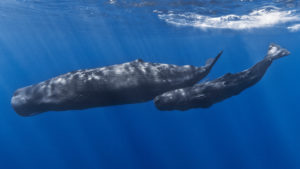Meat is a product that is typically sold by the pound. One pound of ground beef makes about four hamburger patties.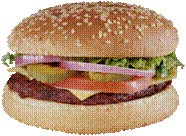Ounces are used to measure lighter objects. A stack of 11 pennies is equal to about one ounce.You can use any of the customary measurement units to describe the weight of something, but it makes more sense to use certain units for certain purposes. For example, it makes more sense to describe the weight of a human being in pounds rather than tons. It makes more sense to describe the weight of a car in tons rather than ounces.

1 pound = 16 ounces

$\frac{16\text{ ounces}}{1\text{ pound}}$

### Converting Between Units of Weight

Four ounces is a typical serving size of meat. Since meat is sold by the pound, you might want to convert the weight of a package of meat from pounds to ounces in order to determine how many servings are contained in a package of meat.

The weight capacity of a truck is often provided in tons. You might need to convert pounds into tons if you are trying to determine whether a truck can safely transport a big shipment of heavy materials.

The table below shows the unit conversions and conversion factors that are used to make conversions between customary units of weight.

Unit Equivalents Conversion Factors (heavier to lighter units of measurement) Conversion Factors(lighter to heavier units of measurement)

1 pound = 16 ounces

$\frac{16\text{ ounces}}{1\text{ pound}}$

$\displaystyle \frac{\text{1 pound}}{\text{16 ounces}}$

1 ton = 2000 pounds

$\frac{2000\text{ pounds}}{1\text{ ton}}$

$\displaystyle \frac{\text{1 ton}}{\text{2000 pounds}}$

You can use the factor label method to convert one customary unit of weight to another customary unit of weight. This method uses conversion factors, which allow you to cancel units to end up with your desired unit of measurement.

Each of these conversion factors is a ratio of equal values, so each conversion factor equals 1. Multiplying a measurement by a conversion factor does not change the size of the measurement at all, since it is the same as multiplying by 1. It just changes the units that you are using to measure it in.

Two examples illustrating the factor label method are shown below.

### Exercises

How many ounces are in $\displaystyle 2\frac{1}{4}$ pounds?

### Example

How many tons is 6,500 pounds?

### Applications of Unit Conversions With Weight

There are times when you need to perform calculations on measurements that are given in different units. To solve these problems, you need to convert one of the measurements to the same unit of measurement as the other measurement.

Think about whether the unit you are converting to is smaller or larger than the unit you are converting from. This will help you be sure that you are making the right computation. You can use the factor label method to make the conversion from one unit to another.

Here is an example of a problem that requires converting between units.

### Example

A municipal trash facility allows a person to throw away a maximum of 30 pounds of trash per week. Last week, 140 people threw away the maximum allowable trash. How many tons of trash did this equal?

### TRY IT NOW

Let’s revisit the coffee price problem that was posed earlier. We can use unit conversion to solve this problem.

### Example

The grocery store sells a 36 ounce canister of ground coffee for $14, and sells bulk coffee for$7 per pound. Which is the better deal?

### TRY IT NOW

The average weight of a northern bluefin tuna is 1,800 pounds. The average weight of a great white shark is $\displaystyle 2\frac{1}{2}$ tons. On average, how much more does a great white shark weigh, in pounds, than a northern bluefin tuna?

### Summary

In the U.S. customary system of measurement, weight is measured in three units: ounces, pounds, and tons. A pound is equivalent to 16 ounces, and a ton is equivalent to 2,000 pounds. While an objects weight can be described using any of these units, it is typical to describe very heavy objects using tons and very light objects using an ounce. Pounds are used to describe the weight of many objects and people.

Often, in order to compare the weights of two objects or people or to solve problems involving weight, you must convert from one unit of measurement to another unit of measurement. Using conversion factors with the factor label method is an effective strategy for converting units and solving problems.

## Units of Capacity

Capacity is the amount of liquid (or other pourable substance) that an object can hold when its full. When a liquid, such as milk, is being described in gallons or quarts, this is a measure of capacity.

Understanding units of capacity can help you solve problems like this: Sven and Johanna were hosting a potluck dinner. They did not ask their guests to tell them what they would be bringing, and three people ended up bringing soup. Erin brought 1 quart, Richard brought 3 pints, and LeVar brought 9 cups. How many cups of soup did they have all together?

There are five main units for measuring capacity in the U.S. customary measurement system. The smallest unit of measurement is a fluid ounce. Ounce is also used as a measure of weight, so it is important to use the word fluid with ounce when you are talking about capacity. Sometimes the prefix fluid is not used when it is clear from the context that the measurement is capacity, not weight.

The other units of capacity in the customary system are the cup, pint, quart, and gallon. The table below describes each unit of capacity and provides an example to illustrate the size of the unit of measurement.

 Fluid Ounce A unit of capacity equal to $\displaystyle \frac{1}{8}$ of a cup. One fluid ounce of water at 62°F weighs about one ounce. The amount of liquid medicine is often measured in fluid ounces.Cup A unit equal to 8 fluid ounces. The capacity of a standard measuring cup is one cup.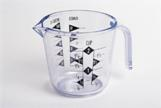Pint A unit equal to 16 fluid ounces, or 2 cups. The capacity of a carton of ice cream is often measured in pints.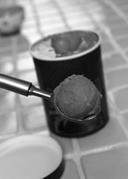Quart A unit equal to 32 fluid ounces, or 4 cups. You often see quarts of milk being sold in the supermarket.Gallon A unit equal to 4 quarts, or 128 fluid ounces. When you fill up your car with gasoline, the price of gas is often listed in dollars per gallon.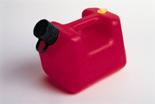You can use any of these five measurement units to describe the capacity of an object, but it makes more sense to use certain units for certain purposes. For example, it makes more sense to describe the capacity of a swimming pool in gallons and the capacity of an expensive perfume in fluid ounces.

### Try It

Sometimes you will need to convert between units of measurement. For example, you might want to express 5 gallons of lemonade in cups if you are trying to determine how many 8-fluid ounce servings the amount of lemonade would yield.

The table below shows some of the most common equivalents and conversion factors for the five customary units of measurement of capacity.

 Unit Equivalents Conversion Factors (heavier to lighter units of measurement) Conversion Factors (lighter to heavier units of measurement) 1 cup = 8 fluid ounces $\displaystyle \frac{\text{1 cup}}{\text{8 fluid ounces}}$ $\displaystyle \frac{\text{8 fluid ounces}}{\text{1 cup}}$ 1 pint = 2 cups $\displaystyle \frac{\text{1 pint}}{2\text{ cups}}$ $\displaystyle \frac{2\text{ cups}}{1\text{ pint}}$ 1 quart = 2 pints $\displaystyle \frac{\text{1 quart}}{2\text{ pints}}$ $\displaystyle \frac{2\text{ pints}}{\text{1 quart}}$ 1 quart = 4 cups $\displaystyle \frac{\text{1 quart}}{4\text{ cups}}$ $\displaystyle \frac{4\text{ cups}}{\text{1 quart}}$ 1 gallon = 4 quarts $\displaystyle \frac{\text{1 gallon}}{4\text{ quarts}}$ $\displaystyle \frac{4\text{ quarts}}{\text{1 gallon}}$ 1 gallon = 16 cups $\displaystyle \frac{1\text{ gallon}}{\text{16 cups}}$ $\displaystyle \frac{\text{16 cups}}{1\text{ gallon}}$

### Converting Between Units of Capacity

As with converting units of length and weight, you can use the factor label method to convert from one unit of capacity to another. An example of this method is shown below.

### Example

How many pints is $\displaystyle 2\frac{3}{4}$ gallons?

### Example

How many gallons is 32 fluid ounces?

### Try It

Find the sum of 4 gallons and 2 pints. Express your answer in cups.

### Applying Unit Conversions

There are times when you will need to combine measurements that are given in different units. In order to do this, you need to convert first so that the units are the same.

Consider the situation posed earlier in this topic.

### Exercises

Sven and Johanna were hosting a potluck dinner. They did not ask their guests to tell them what they would be bringing, and three people ended up bringing soup. Erin brought 1 quart, Richard brought 3 pints, and LeVar brought 9 cups. How much soup did they have total?

### Exercises

Natasha is making lemonade to bring to the beach. She has two containers. One holds one gallon and the other holds 2 quarts. If she fills both containers, how many cups of lemonade will she have?

Another way to work the problem above would be to first change 1 gallon to 16 cups and change 2 quarts to 8 cups. Then add: $16+8=24$ cups.

In the following video we provide another example of using unit conversions to solve a problem.  We show how to find the number of lemons needed to make a pie, given that each lemon yields about 4 tablespoons of juice.

### Try It

Alan is making chili. He is using a recipe that makes 24 cups of chili. He has a 5-quart pot and a 2-gallon pot and is trying to determine whether the chili will all fit in one of these pots. Which of the pots will fit the chili?

In the following ~10 minute video, we provide a mini-lesson that covers US measurements for length, weight, and capacity, and how to convert between larger and smaller units for each type. This is a good summary of the concepts covered in the US Units of Measurement section of this module.

### Summary

There are five basic units for measuring capacity in the U.S. customary measurement system. These are the fluid ounce, cup, pint, quart, and gallon. These measurement units are related to one another, and capacity can be described using any of the units. Typically, people use gallons to describe larger quantities and fluid ounces, cups, pints, or quarts to describe smaller quantities. Often, in order to compare or to solve problems involving the amount of liquid in a container, you need to convert from one unit of measurement to another.